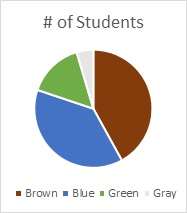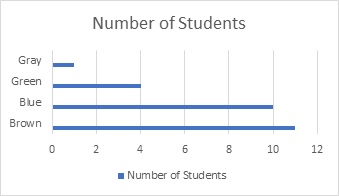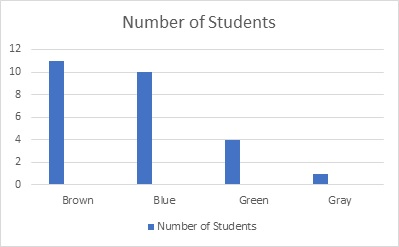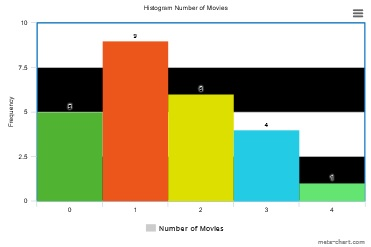# Mathematics Exercise Sample Assignment

HOMEWORK

BY VAUGHN FIELDINGCHILES

Lane Exercise.

#8.

1. Pie Chart
2.3. Horizontal Bar Chart
4.5. Vertical Bar Graph
6.7. A Frequency Table with the relative frequency of each eye color
 Eye Color Frequency Relative Frequency Cumulative Relative Frequency Brown 11 11/26= 0.423 0.423 Blue 10 10/26= 0.385 0.808 (.385+.423) Green 4 4/26= 0.154 0.962 (.154+.808) Gray 1 1/26= 0.038 1 (.038+.962)

9. Which of the box plots on the graph has a large positive skew? Answer B. On the reading assignment page 98 talks about how the distribution with a positive skew would have a longer whisker in the positive direction. The distance from the median to the upper adjacent line is greater when measured and compared to the distance from the lower adjacent line to the median.

Which has a large negative skew? Answer C. Based off my previous answer the same rule applies however, this is view when the situation is vice-versa.

6. You had calculated the following measures of central tendency: the mean, the median, and the mean trimmed 25%. Which of these measures of central tendency will change when you correct the recording error? The mean and the mean trimmed would change.

15.2 18.8 19.3 19.7 20.2 21.8 22.1 29.4

Mean = 15.2 + 18.8 + 19.3 + 19.7 + 20.2 + 21.8 + 22.1 + 29.4 = 166.5 166.5 / 8 = 20.8125

Median = (19.7 + 20.2) / 2 = 19.95

Mean Trimmed 25% = remove the lower 12.5% of recordings as well as the upper 12.5% of the recordings.

12.5% = 0.125 x 8 = 1

Remove 1 recording time from the upper set of data and remove 1 recording time from the lower set of data:

15.2 18.8 19.3 19.7 20.2 21.8 22.1 29.4

18.8+19.3+19.7+20.2+21.8+22.1 = 121.9 121.9 / 6 = 20.3167

8. You know the minimum, the maximum, and the 25th, 50th, and 75th percentiles of a distribution. Which of the following measures of central tendency or variability can you determine? The mean, trimean, range, and the interquartile range.

Median = the 50th percentile of a distribution is also equal to the median

Trimean = the weighted average of the 25th percentile, 2 times the 50th percentile and the 75th percentile.

Range = maximum - minimum Interquartile range = (75th percentile – 25th percentile)

30.(AT) What is the mean number of correct responses of the participants after taking the placebo (0 mg/kg)?  M = ΣX/N (300/5= 60)

For the ADHD Treatment (AT) case study, what is the mean number of correct responses of the participants after taking the placebo (0 mg/kg)?  mean = sum of data/# of data = 954/24 = 39.75

31.(AT) What are the standard deviation and the interquartile range of the d0 condition?  SD = 11.31

Interquartile range = 12

Illowski Ex: 78,80

78.

 # of Movies Frequency Relative Frequency Cumulative Relative Frequency 0 5 5/25=0.2 0.2 1 9 9/25=0.36 0.2+0.36=0.56 2 6 6/25=0.24 0.56+0.24=0.8 3 4 4/25=0.16 0.16+0.8=0.98 4 1 1/25=0.04 0.98+0.04=1.0281. If the data were collected by asking the first 111 people who entered the store, then the type of sampling is: